Home » 40 Questions to test your skill in Python for Data Science

# 40 Questions to test your skill in Python for Data Science

Python is increasingly becoming popular among data science enthusiasts, and for right reasons. It brings the entire ecosystem of a general programming language. So you can not only transform and manipulate data, but you can also create strong pipelines and machine learning workflows in a single ecosystem.

At Analytics Vidhya, we love Python. Most of us use Python as our preferred tool for machine learning. Not only this, if you want to learn Deep Learning, Python clearly has the most mature ecosystem among all other languages.

If you are learning Python for Data Science, this test was created to help you assess your skill in Python. This test was conducted as part of DataFest 2017. Close to 1,300 people participated in the test with more than 300 people taking this test.

Below are the distribution scores of the people who took the test: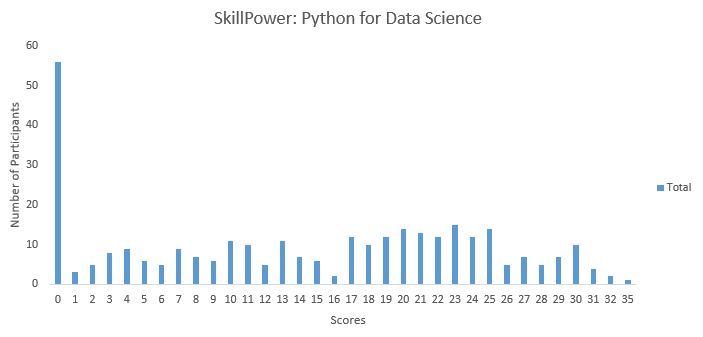You can access the final scores here. Here are a few statistics about the distribution.

Mean Score: 14.16

Median Score: 15

Mode Score: 0Question Context 1

You must have seen the show “How I met your mother”. Do you remember the game where they played, in which each person drinks a shot whenever someone says “but, um”. I thought of adding a twist to the game. What if you could use your technical skills to play this game?

To identify how many shots a person is having in the entire game, you are supposed to write a code.

Below is the subtitle sample script.

Note: Python regular expression library has been imported as re.

```txt = '''450
00:17:53,457 --> 00:17:56,175
Okay, but, um,
thanks for being with us.

451
00:17:56,175 --> 00:17:58,616
But, um, if there's any
college kids watching,

452
00:17:58,616 --> 00:18:01,610
But, um, but, um, but, um,
but, um, but, um,

453
00:18:01,610 --> 00:18:03,656
We have to drink, professor.```
```454
00:18:03,656 --> 00:18:07,507
It's the rules.
She said "But, um"

455
00:18:09,788 --> 00:18:12,515
But, um, but, um, but, um...
god help us all.
'''```

1) Which of the following codes would be appropriate for this task?

A) len(re.findall(‘But, um’, txt))

B) re.search(‘But, um’, txt).count()

C) len(re.findall(‘[B,b]ut, um’, txt))

D) re.search(‘[B,b]ut, um’, txt)).count()

Solution: (C)

You have to find both capital and small versions of “but” So option C is correct.

Question Context 2

Suppose you are given the below string

[email protected],aa,Owner,2014
[email protected],bb,Member,2015
[email protected],cc,Member,2017
[email protected],dd,Member,2016
[email protected],ee,Member,2020
“””

In order to extract only the domain names from the email addresses from the above string (for eg. “aaa”, “bbb”..) you write the following code:

```for i in re.finditer('([a-zA-Z]+)@([a-zA-Z]+).(com)', str):
print i.group(__)```

2) What number should be mentioned instead of “__” to index only the domains?

Note: Python regular expression library has been imported as re.

A) 0

B) 1

C) 2

D) 3

Solution: (C)

Read syntax of regular expression re.

Question Context 3

Your friend has a hypothesis – “All those people who have names ending with the sound of “y” (Eg: Hollie) are intelligent people.” Please note: The name should end with the sound of ‘y’ but not end with alphabet ‘y’.

Now you being a data freak, challenge the hypothesis by scraping data from your college’s website. Here’s data you have collected.

 Name Marks Andy 0 Mandi 10 Sandy 20 Hollie 18 Molly 19 Dollie 15

You want to make a list of all people who fall in this category. You write following code do to the same:

```temp = []
for i in re.finditer(pattern, str):
temp.append(i.group(1))```

3) What should be the value of “pattern” in regular expression?

Note: Python regular expression library has been imported as re.

A) pattern = ‘(i|ie)(,)’

B) pattern = ‘(i\$|ie\$)(,)’

C) pattern = ‘([a-zA-Z]+i|[a-zA-Z]+ie)(,)’

D) None of these

Solution: (B)

You have to find the pattern the end in either “i” or “ie”. So option B is correct.

Question Context 4

Assume, you are given two lists:

a = [1,2,3,4,5]

b = [6,7,8,9]

The task is to create a list which has all the elements of a and b in one dimension.

Output:

a = [1,2,3,4,5,6,7,8,9]

4) Which of the following option would you choose?

A) a.append(b)

B) a.extend(b)

C) Any of the above

D) None of these

Solution: (B)

Option B is correct

5) You have built a machine learning model which you wish to freeze now and use later. Which of the following command can perform this task for you?

Note: Pickle library has been imported as pkl.

A) push(model, “file”)

B) save(model, “file”)

C) dump(model, “file”)

D) freeze(model, “file”)

Solution: (C)

Option C is correct

Question Context 6

We want to convert the below string in date-time value:

```import time
str = '21/01/2017'
datetime_value = time.strptime(str,date_format)```

6) To convert the above string, what should be written in place of date_format?

A) “%d/%m/%y”

B) “%D/%M/%Y”

C) “%d/%M/%y”

D) “%d/%m/%Y”

Solution: (D)

Option D is correct

Question Context 7

I have built a simple neural network for an image recognition problem. Now, I want to test if I have assigned the weights & biases for the hidden layer correctly. To perform this action, I am giving an identity matrix as input. Below is my identity matrix:

A =  [ 1, 0, 0
0, 1, 0
0, 0, 1]

7) How would you create this identity matrix in python?

Note: Library numpy has been imported as np.

A) np.eye(3)

B) identity(3)

C) np.array([1, 0, 0], [0, 1, 0], [0, 0, 1])

D) All of these

Solution: (A)

Option B does not exist (it should be np.identity()). And option C is wrong, because the syntax is incorrect. So the answer is option A

8) To check whether the two arrays occupy same space, what would you do?

I have two numpy arrays “e” and “f”.

You get the following output when you print “e” & “f”

```print e
[1, 2, 3, 2, 3, 4, 4, 5, 6]
print f
[[1, 2, 3], [2, 3, 4], [4, 5, 6]]```

When you change the values of the first array, the values for the second array also changes. This creates a problem while processing the data.

For example, if you set the first 5 values of e as 0; i.e.

```print e[:5]

0
```

the final values of e and f are

```print e

[0, 0, 0, 0, 0, 4, 4, 5, 6]

print f

[[0, 0, 0], [0, 0, 4], [4, 5, 6]]```

You surmise that the two arrays must have the same space allocated.

A) Check memory of both arrays, if they match that means the arrays are same.

B) Do “np.array_equal(e, f)” and if the output is “True” then they both are same

C) Print flags of both arrays by e.flags and f.flags; check the flag “OWNDATA”. If one of them is False, then both the arrays have same space allocated.

D) None of these

Solution: (C)

Option C is correct

Question Context 9

Suppose you want to join train and test dataset (both are two numpy arrays train_set and test_set) into a resulting array (resulting_set) to do data processing on it simultaneously. This is as follows:

```train_set = np.array([1, 2, 3])
test_set = np.array([[0, 1, 2], [1, 2, 3]])
resulting_set --> [[1, 2, 3], [0, 1, 2], [1, 2, 3]]```

9) How would you join the two arrays?

Note: Numpy library has been imported as np

A) resulting_set = train_set.append(test_set)

B) resulting_set = np.concatenate([train_set, test_set])

C) resulting_set = np.vstack([train_set, test_set])

D) None of these

Solution: (C)

Both option A and B would do horizontal stacking, but we would like to have vertical stacking. So option C is correct

Question Context 10

Suppose you are tuning hyperparameters of a random forest classifier for the Iris dataset.

 Sepal_length Sepal_width Petal_length Petal_width Species 4.6 3.2 1.4 0.2 Iris-setosa 5.3 3.7 1.5 0.2 Iris-setosa 5.0 3.3 1.4 0.2 Iris-setosa 7.0 3.2 4.7 1.4 Iris-versicolor 6.4 3.2 4.5 1.5 Iris-versicolor

10) What would be the best value for “random_state (Seed value)”?

A) np.random.seed(1)

B) np.random.seed(40)

C) np.random.seed(32)

D) Can’t say

Solution: (D)

There is no best value for seed. It depends on the data.

Question 11

While reading a csv file with numpy, you want to automatically fill missing values of column “Date_Of_Joining” with date “01/01/2010”.

 Name Age Date_Of_Joining Total_Experience Andy 20 01/02/2013 0 Mandy 30 01/05/2014 10 Sandy 10 0 Bandy 40 01/10/2009 20

11) Which command will be appropriate to fill missing value while reading the file with numpy?

Note: numpy has been imported as np

A) filling_values = (“-“, 0, 01/01/2010, 0)
temp = np.genfromtxt(filename, filling_values=filling_values)

B) filling_values = (“-“, 0, 01/01/2010, 0)

C) filling_values = (“-“, 0, 01/01/2010, 0)
temp = np.gentxt(filename, filling_values=filling_values)

D) None of these

Solution: (A)

Option A is correct

12) How would you import a decision tree classifier in sklearn?

A) from sklearn.decision_tree import DecisionTreeClassifier

B) from sklearn.ensemble import DecisionTreeClassifier

C) from sklearn.tree import DecisionTreeClassifier

D) None of these

Solution: (C)

Option C is correct

13) You have uploaded the dataset in csv format on google spreadsheet and shared it publicly. You want to access it in python, how can you do this?

Note: Library StringIO has been imported as StringIO.

```A)
```B)

```

C)

```link = https://docs.google.com/spreadsheets/d/...source = StringIO(requests.get(link).content))

D) None of these

Solution: (A)

Option A is correct

Question Context 14

Imagine, you have a dataframe train file with 2 columns & 3 rows, which is loaded in pandas.

import pandas as pd

`train  = pd.DataFrame({'id':[1,2,4],'features':[["A","B","C"],["A","D","E"],["C","D","F"]]})`

Now you want to apply a lambda function on “features” column:

`train['features_t'] = train["features"].apply(lambda x: " ".join(["_".join(i.split(" ")) for i in x]))`

14) What will be the output of following print command?

`print train['features_t']`

A)

0    A B C
1    A D E
2    C D F

B)

0    AB

2    CDF

C) Error
D) None of these

Solution: (A)

Option A is correct

Question Context 15

We have a multi-class classification problem for predicting quality of wine on the basis of its attributes. The data is loaded in a dataframe “df”

 fixed acidity volatile acidity citric acid residual sugar chlorides free sulfur dioxide total sulfur dioxide density pH sulphates Alcohol quality 0 7.4 0.70 0.00 1.9 0.076 11 34 0.9978 3.51 0.56 9.4 5 1 7.8 0.88 0.00 2.6 0.098 25 67 0.9968 3.20 0.68 9.8 5 2 7.8 0.76 0.04 2.3 0.092 15 54 0.9970 3.26 0.65 9.8 5 3 11.2 0.28 0.56 1.9 0.075 17 60 0.9980 3.16 0.58 9.8 6 4 7.4 0.70 0.00 1.9 0.076 11 34 0.9978 3.51 0.56 9.4 5

The quality column currently has values 1 to 10, but we want to substitute this by a binary classification problem. You want to keep the threshold for classification to 5, such that if the class is greater than 5, the output should be 1, else output should be 0.

Note: Numpy has been imported as np and dataframe is set as df.

A)

```Y = df[quality].values
Y = np.array([1 if y >= 6 else 0 for y in Y])```

B)

```Y = df[quality].values()
Y = np.array([0 if y >= 6 else 1 for y in Y])```

C)

```Y = df[quality]
Y = np.array([0 if y >= 6 else 1 for y in Y])```

D)None of these

Solution: (A)

Option A is correct

Question Context 16

Suppose we make a dataframe as

```df = pd.DataFrame(['ff', 'gg', 'hh', 'yy'],
[24, 12, 48, 30],
columns = ['Name', 'Age'])```

16) What is the difference between the two data series given below?

1. df[‘Name’] and
2. df.loc[:, ‘Name’]

Note: Pandas has been imported as pd

A) 1 is view of original dataframe and 2 is a copy of original dataframe.

B) 2 is view of original dataframe and 1 is a copy of original dataframe.

C) Both are copies of original dataframe.

D) Both are views of original dataframe

Solution: (B)

Option B is correct. Refer the official docs of pandas library.

Question Context 17

Consider a function “fun” which is defined below:

```def fun(x):
x = 5
return x```

Now you define a list which has three numbers in it.

g = [10,11,12]

17) Which of the following will be the output of the given print statement:

`print  fun(g), g`

A) [5, 11, 12] [5, 11, 12]

B) [5, 11, 12] [10, 11, 12]

C) [10, 11, 12] [10, 11, 12]

D) [10, 11, 12] [5, 11, 12]

Solution: (A)

Option A is correct

Question Context 18

Sigmoid function is usually used for creating a neural network activation function. A sigmoid function is denoted as

```def sigmoid(x):
return (1 / (1 + math.exp(-x)))```

18) It is necessary to know how to find the derivatives of sigmoid, as it would be essential for backpropagation. Select the option for finding derivative?

A)

```import scipy

Dv = scipy.misc.derive(sigmoid)```

B)

```from sympy import *

x = symbol(x)

y = sigmoid(x)

Dv = y.differentiate(x)```

C)

`Dv = sigmoid(x) * (1 - sigmoid(x))`

D) None of these

Solution: (C)

Option C is correct

Question Context 19

Suppose you are given a monthly data and you have to convert it to daily data.

For example,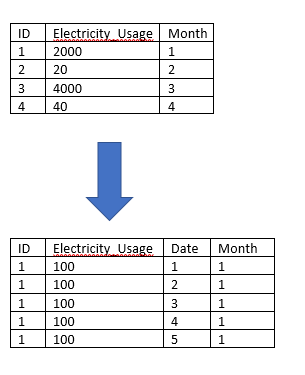For this, first you have to expand the data for every month (considering that every month has 30 days)

19) Which of the following code would do this?

Note: Numpy has been imported as np and dataframe is set as df.

A) new_df = pd.concat([df]*30, index = False)

B) new_df = pd.concat([df]*30, ignore_index=True)

C) new_df = pd.concat([df]*30, ignore_index=False)

D) None of these

Solution: (B)

Option B is correct

Context: 20-22

Suppose you are given a dataframe df.

`df = pd.DataFrame({'Click_Id':['A','B','C','D','E'],'Count':[100,200,300,400,250]})`

20) Now you want to change the name of the column ‘Count’ in df to ‘Click_Count’. So, for performing that action you have written the following code.

`df.rename(columns = {'Count':'Click_Count'})`

What will be the output of print statement below?

`print df.columns`

Note: Pandas library has been imported as pd.

A) [‘Click_Id’, ‘Click_Count’]

B) [‘Click_Id’, ‘Count’]

C) Error

D) None of these

Solution: (B)

Option B is correct

Context: 20-22

Suppose you are given a data frame df.

`df = pd.DataFrame({'Click_Id':['A','B','C','D','E'],'Count':[100,200,300,400,250]})`

21) In many data science projects, you are required to convert a dataframe into a dictionary. Suppose you want to convert “df” into a dictionary such that ‘Click_Id’ will be the key and ‘Count’ will be the value for each key. Which of the following options will give you the desired result?

Note: Pandas library has been imported as pd

A) set_index(‘Click_Id’)[‘Count’].to_dict()

B) set_index(‘Count’)[‘Click_Id’].to_dict()

C) We cannot perform this task since dataframe and dictionary are different data structures

D) None of these

Solution: (A)

Option A is correct

22) In above dataframe df. Suppose you want to assign a df to df1, so that you can recover original content of df in future using df1 as below.

`df1 = df`

Now you want to change some values of “Count” column in df.

`df.loc[df.Click_Id == 'A', 'Count'] += 100`

Which of the following will be the right output for the below print statement?

`print df.Count.values,df1.Count.values`

Note: Pandas library has been imported as pd.

A) [200 200 300 400 250] [200 200 300 400 250]

B) [100 200 300 400 250] [100 200 300 400 250]

C) [200 200 300 400 250] [100 200 300 400 250]

D) None of these

Solution: (A)

Option A is correct

23) You write a code for preprocessing data, and you notice it is taking a lot of time. To amend this, you put a bookmark in the code so that you come to know how much time is spent on each code line. To perform this task, which of the following actions you would take?

1. You put bookmark as time.sleep() so that you would know how much the code has “slept” literally
2. You put bookmark as time.time() and check how much time elapses in each code line
3. You put bookmark as datetime.timedelta(), so that you would find out differences of execution times
4. You copy whole code in an Ipython / Jupyter notebook, with each code line as a separate block and write magic function %%timeit in each block

A) 1 & 2

B) 1,2 & 3

C) 1,2 & 4

D) All of the above

Solution: (C)

Option C is correct

24) How would you read data from the file using pandas by skipping the first three lines?

Note: pandas library has been imported as pd In the given file (email.csv), the first three records are empty.

```,,,

,,,

,,,

[email protected],aa,Owner,2014

[email protected],bb,Member,2015

[email protected],cc,Member,2017

[email protected],dd,Member,2016```

D) None of these

Solution: (B)

Option B is correct

25) What should be written in-place of “method” to produce the desired outcome?

Given below is dataframe “df”: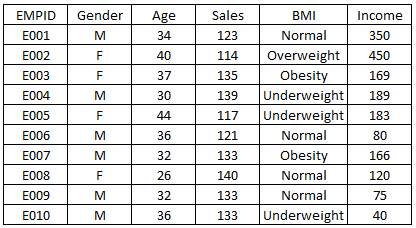Now, you want to know whether BMI and Gender would influence the sales.

For this, you want to plot a bar graph as shown below: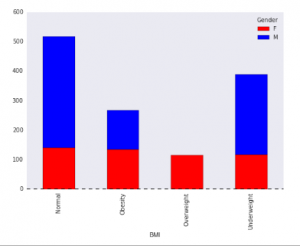The code for this is:

```var = df.groupby(['BMI','Gender']).Sales.sum()

var.unstack().plot(kind='bar', method,  color=['red','blue'], grid=False)```

A) stacked=True

B) stacked=False

C) stack=False

D) None of these

Solution: (A)

It’s a stacked bar chart.

26) Suppose, you are given 2 list – City_A and City_B.

City_A = [‘1′,’2′,’3′,’4’]

City_B = [‘2′,’3′,’4′,’5’]

In both cities, some values are common. Which of the following code will find the name of all cities which are present in “City_A” but not in “City_B”.

A) [i for i in City_A if i not in City_B]

B) [i for i in City_B if i not in City_A]

C) [i for i in City_A if i in City_B]

D) None of these

Solution: (A)

Option A is correct

Question Context 27

Suppose you are trying to read a file “temp.csv” using pandas and you get the following error.

```Traceback (most recent call last):
File "<input>", line 1, in<module>
UnicodeEncodeError: 'ascii' codec can't encode character.```

27) Which of the following would likely correct this error?

Note: pandas has been imported as pd

D) None of these

Solution: (C)

Option C is correct, because encoding should be ‘utf-8’

28) Suppose you are defining a tuple given below:

tup = (1, 2, 3, 4, 5 )

Now, you want to update the value of this tuple at 2nd index to 10. Which of the following option will you choose?

A) tup(2) = 10

B) tup = 10

C) tup{2} = 10

D) None of these

Solution: (D)

A tuple cannot be updated.

29) You want to read a website which has url as “www.abcd.org”. Which of the following options will perform this task?

A) urllib2.urlopen(www.abcd.org)

B) requests.get(www.abcd.org)

C) Both A and B

D) None of these

Solution: (C)

Option C is correct

Question Context 30

Suppose you are given the below web page

```html_doc = “””
<!DOCTYPE html>
<htmllang="en">
<metacharset="utf-8">
<metaname="viewport" content="width=device-width">
<title>udacity/deep-learning: Repo for the Deep Learning Nanodegree Foundations program.</title>
<metaproperty="fb:app_id" content="1401488693436528">
...

“””```

30) To read the title of the webpage you are using BeautifulSoup. What is the code for this?

Hint: You have to extract text in title tag

1. from bs4 import BeautifulSoup
soup =BeautifulSoup(html_doc,’html.parser’)
print soup.title.name
2. from bs4 import BeautifulSoup
soup =BeautifulSoup(html_doc,’html.parser’)
print soup.title.string
3. from bs4 import BeautifulSoup
soup=BeautifulSoup(html_doc,’html.parser’)
print soup.title.get_text
4. None of these

Solution: (B)

Option B is correct
Question Context 31

Imagine, you are given a list of items in a DataFrame as below.

D = [‘A’,’B’,’C’,’D’,’E’,’AA’,’AB’]

Now, you want to apply label encoding on this list for importing and transforming, using LabelEncoder.

```from sklearn.preprocessing import LabelEncoder

le = LabelEncoder()```

31) What will be the output of the print statement below ?

`print le.fit_transform(D)`
1. array([0, 2, 3, 4, 5, 6, 1])
2. array([0, 3, 4, 5, 6, 1, 2])
3. array([0, 2, 3, 4, 5, 1, 6])
4. Any of the above

Solution: (D)

Option D is correct

32) Which of the following will be the output of the below print statement?

`print df.val == np.nan`

Assume, you have defined a data frame which has 2 columns.

```import numpy as np

df = pd.DataFrame({'Id':[1,2,3,4],'val':[2,5,np.nan,6]})```

A) 0   False
1    False
2   False
3    False

B)  0    False
1    False
2    True
3    False

C) 0 True
1    True
2    True
3    True

D) None of these

Solution: (A)

Option A is correct

33) Suppose the data is stored in HDFS format and you want to find how the data is structured. For this, which of the following command would help you find out the names of HDFS keys?

Note: HDFS file has been loaded by h5py as hf.

A) hf.key()

B) hf.key

C) hf.keys()

D) None of these

Solution: (C)

Option C is correct

Question Context 34

You are given reviews for movies below:

reviews = [‘movie is unwatchable no matter how decent the first half is  . ‘, ‘somewhat funny and well  paced action thriller that has jamie foxx as a hapless  fast  talking hoodlum who is chosen by an overly demanding’, ‘morse is okay as the agent who comes up with the ingenious plan to get whoever did it at all cost .’]

Your task is to find sentiments from the review above. For this, you first write a code to find count of individual words in all the sentences.

```counts = Counter()

for i in range(len(reviews)):
for word in reviews[i].split(value):
counts[word] += 1```

34)What value should we split on to get individual words?

1. ‘ ‘
2. ‘,’
3. ‘.’
4. None of these

Solution: (A)

Option A is correct

35) How to set a line width in the plot given below?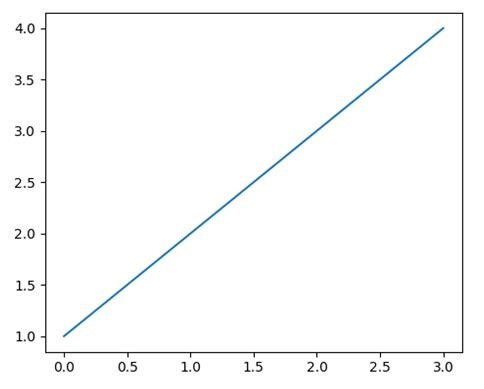For the above graph, the code for producing the plot was

```import matplotlib.pyplot as plt
plt.plot([1,2,3,4])
plt.show()```
1. In line two, write plt.plot([1,2,3,4], width=3)
2. In line two, write plt.plot([1,2,3,4], line_width=3
3. In line two, write plt.plot([1,2,3,4], lw=3)
4. None of these

Solution: (C)

Option C is correct

36) How would you reset the index of a dataframe to a given list? The new index is given as:

new_index=[‘Safari’,’Iceweasel’,’Comodo Dragon’,’IE10′,’Chrome’]

Note: df is a pandas dataframe

 http_status response_time Firefox 200 0.04 Chrome 200 0.02 Safari 404 0.07 IE10 404 0.08 Konqueror 301 1.00

A) df.reset_index(new_index,)

B) df.reindex(new_index,)

C) df.reindex_like(new_index,)

D) None of these

Solution: (A)

Option A is correct

37) Determine the proportion of passengers survived based on their passenger class.

 PassengerId Survived Pclass Name Sex Age SibSp Parch Ticket Fare Cabin Embarked 0 1 0 3 Braund, Mr. Owen Harris male 22.0 1 0 A/5 21171 7.2500 NaN S 1 2 1 1 Cumings, Mrs. John Bradley (Florence Briggs Th… female 38.0 1 0 PC 17599 71.2833 C85 C 2 3 1 3 Heikkinen, Miss. Laina female 26.0 0 0 STON/O2. 3101282 7.9250 NaN S 3 4 1 1 Futrelle, Mrs. Jacques Heath (Lily May Peel) female 35.0 1 0 113803 53.1000 C123 S 4 5 0 3 Allen, Mr. William Henry male 35.0 0 0 373450 8.0500 NaN S

1. crosstab(df_train[‘Pclass’], df_train[‘Survived’])
2. proportion(df_train[‘Pclass’], df_train[‘Survived’])
3. crosstab(df_train[‘Survived’], df_train[‘Pclass’])
4. None of these

Solution: (A)

Option A is correct

38) You want to write a generic code to calculate n-gram of the text. The 2-gram of this sentence would be [[“this, “is”], [“is”, “a”], [“a, “sample”], [“sample”, “text”]]

Which of the following code would be correct?

For a given a sentence:
‘this is a sample text’.

1. def generate_ngrams(text, n):
words = text.split(‘\n’)
output = []
for i in range(len(words)-n+1):
append(words[i+1:i+n])
return output
2. def generate_ngrams(text, n):
words = text.split()
output = []
for i in range(len(words)-n+1):
append(words[i:i+n])
return output
3. def generate_ngrams(text, n):
words = text.split()
output = []
for i in range(len(words)-n+1):
append(words[i+1:i+n])
return output
4. None of these

Solution: (B)

Option B is correct

39) Which of the following code will export dataframe (df) in CSV file, encoded in UTF-8 after hiding index & header labels.

4. None of these

Solution: (C)

Option C is correct

40) Which of the following is a correct implementation of mean squared error (MSE) metric?

Note: numpy library has been imported as np.

1. def MSE(real_target, predicted_target):
return np.mean((np.square(real_target) – np.square(predicted_target)))
2. def MSE(real_target, predicted_target):
return np.mean((real_target – predicted_target)**2)
3. def MSE(real_target, predicted_target):
return np.sqrt(np.mean((np.square(real_target) – np.square(predicted_target))))
4. None of the above

Solution: (B)

Option B is correct

### End Notes

If you are learning Python, make sure you go through the test above. It will not only help you assess your skill. You can also see where you stand among other people in the community. If you have any questions or doubts, feel free to post them below.

### Learn, compete, hack and get hired!

You can also read this article on our Mobile APP•Lia says:

For Question Context 7:

np.identity(3), which is option (B) does exist: https://docs.scipy.org/doc/numpy/reference/generated/numpy.identity.html
But option (A) seems to be incorrect as we’ve got to write np.eye(3), don’t we?

•Faizan Shaikh says:

Thank you for letting us know the typo. Actually option B does not exist because it should have been np.identity(), whereas it should have been np.eye() in option A.

•issac says:

For Question Context 16：
•Faizan Shaikh says: